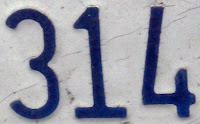## Tuesday, March 30, 2010

### 314

314 = 2 x 157.

314 has a representation as a sum of two squares: 314 = 52 + 172.

314 is the smallest number that can be written as the sum of three positive squares in six ways: 314 = 172 + 42 + 32 = 162 + 72 + 32 = 152 + 82 + 52 = 132 + 92 + 82 = 132 + 122 + 12 = 132 + 92 + 82.

314 is 222 in base 12.

314 is the only number that together with its sixth power, 958468597212736, has each digit from 1 to 9 occurring exactly twice.314 is the telephone area code for St. Louis, Missouri.